# Hausdorffness is closure-local

This article gives the statement, and possibly proof, of a topological space property (i.e., Hausdorff space) satisfying a topological space metaproperty (i.e., closure-local property of topological spaces)
View all topological space metaproperty satisfactions | View all topological space metaproperty dissatisfactions
Get more facts about Hausdorff space |Get facts that use property satisfaction of Hausdorff space | Get facts that use property satisfaction of Hausdorff space|Get more facts about closure-local property of topological spaces

## Statement

Suppose$X$ is a topological space such that for any point$x \in X$, there exists an open subset$U \ni x$ such that the closure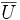$\overline{U}$ is a Hausdorff space. Then,$X$ is also a Hausdorff space.

## Facts used

1. Openness is transitive

## Proof

Given: A topological space$X$ such that for any point$x \in X$, there exists an open subset$U \ni x$ such that the closure$\overline{U}$ is a Hausdorff space. We are given two distinct points$x,y \in X$.

To prove: We can find disjoint open subsets$V,W$ of$X$ such that$V \ni x, W \ni y$.

Proof:

Step no. Assertion/construction Facts used Given data used Previous steps used Explanation
1 There exists an open subset$U \ni x$ such that the closure$\overline{U}$ is a Hausdorff space. given data directly used direct
2 If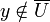$y \notin \overline{U}$, then we can take$V = U$ and$W = X \setminus \overline{U}$. Step (1)
3 If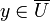$y \in \overline{U}$, there exist open subsets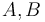$A,B$ of$\overline{U}$ such that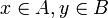$x \in A, y \in B$ and$A \cap B$ is empty.
4 Continuing with the assumption and notation from Step (3), then there exists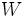$W$ open in$X$ such that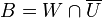$B = W \cap \overline{U}$. In particular,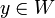$y \in W$. definition of subspace topology Step (3)
5 Continuing with the assumption and notation from Step (3), the set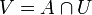$V = A \cap U$ is open in$X$ and contains$x$. Fact (1) Steps (1), (3) By definition of subspace topology going from$\overline{U}$ to$U$,$V$ is open in$U$. Thus, by Fact (1),$V$ is open in$X$. Also,$x \in V$ because$x \in A$ (Step (3)) and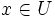$x \in U$ (Step (1)).
6 Continuing with the assumption and notation from Steps (3)-(5), the sets$V$ and$W$ are disjoint. Steps (3), (4) ,(5)$V$ is contained in$U$ by Step (5) and hence in$\overline{U}$. So,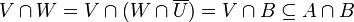$V \cap W = V \cap (W \cap \overline{U}) = V \cap B \subseteq A \cap B$.$A$ and$B$ are disjoint by Step (3), hence the intersection is trivial.
7$V,W$ are the desired disjoint open subsets. Steps (4), (5), (6) Step-combination direct